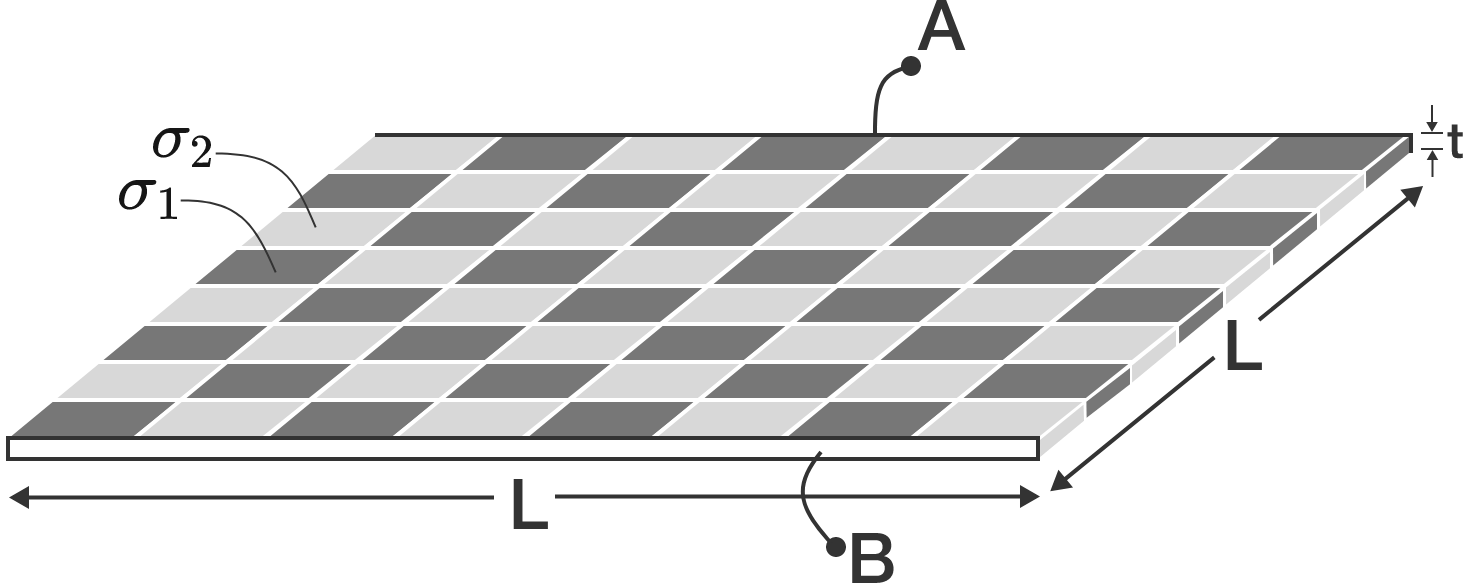# Checkered CapacitorA parallel plate capacitor, with plates $\textbf{A}$ and $\textbf{B}$ of equal dimensions $t \times L$ at a distance of $L$, is filled with square tiles of dielectric to make a chess-board-like capacitor, as shown in the picture above.

Dielectric constant of the dark tile is $\sigma_1,$ that of the light tile is $\sigma_2,$ and $\epsilon_0$ is the permittivity of free space. All the dielectric tiles are square cuboids of thickness $t$.

Find the capacitance of this capacitor.


Details and Assumptions:

• Assume that the electric field varies between the plates like an ideal parallel plate capacitor.
×